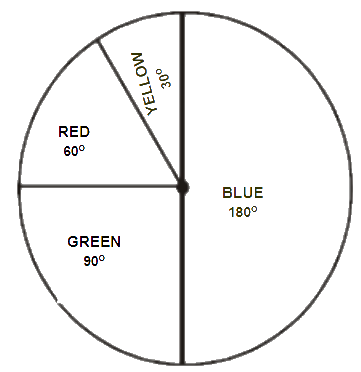# Ex.5.2 Q3 Data Handling Solutions - NCERT Maths Class 8

Go back to  'Ex.5.2'

## Question

Draw a pie chart showing the following information. The table shows the colours preferred by a group of people.

 Colours No. of People Blue $$18$$ Green $$9$$ Red 6 Yellow $$3$$ Total $$36$$

Video Solution
Data Handling
Ex 5.2 | Question 3

## Text Solution

What is known?

Distribution of the colours preferred by a group of people

What is unknown?

Pie chart of the given information.

Reasoning:

A circle graph or pie chart shows the relationship between a whole and its parts.

Steps:

Here, Total angle $$= 360^\circ$$ and Total number of people $$= 36$$

The central angle for each colours can be calculated as below:

 Colours No. of People In Fraction Central Angles Blue $$18$$ \begin{align}\frac{{18}}{{36}} = \frac{1}{2}\end{align} \begin{align}\frac{1}{2} \times {360^{\rm{o}}} = {180^{\rm{o}}}\end{align} Green $$9$$ \begin{align}\frac{9}{{36}} = \frac{1}{4}\end{align} \begin{align}\frac{1}{4} \times {360^{\rm{o}}} = {90^{\rm{o}}}\end{align} Red $$6$$ \begin{align}\frac{6}{{36}} = \frac{1}{6}\end{align} \begin{align}\frac{1}{6} \times {360^{\rm{o}}} = {60^{\rm{o}}}\end{align} Yellow $$3$$ \begin{align}\frac{3}{{36}} = \frac{1}{{12}}\end{align} \begin{align}\frac{1}{{12}} \times {360^{\rm{o}}} = {30^{\rm{o}}}\end{align}Learn from the best math teachers and top your exams

• Live one on one classroom and doubt clearing
• Practice worksheets in and after class for conceptual clarity
• Personalized curriculum to keep up with school